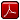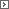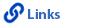Mathematics Grade 8Printable Version (pdf)Course Introduction

Core Standards of the Course

Strand: MATHEMATICAL PRACTICES (8.MP)
The Standards for Mathematical Practice in Eighth Grade describe mathematical habits of mind that teachers should seek to develop in their students. Students become mathematically proficient in engaging with mathematical content and concepts as they learn, experience, and apply these skills and attitudes (Standards 8.MP.1-8).

Standard 8.MP.1
Make sense of problems and persevere in solving them. Explain the meaning of a problem and look for entry points to its solution. Analyze givens, constraints, relationships, and goals. Make conjectures about the form and meaning of the solution, plan a solution pathway, and continually monitor progress asking, "Does this make sense?" Consider analogous problems, make connections between multiple representations, identify the correspondence between different approaches, look for trends, and transform algebraic expressions to highlight meaningful mathematics. Check answers to problems using a different method.

Standard 8.MP.2
Reason abstractly and quantitatively. Make sense of the quantities and their relationships in problem situations. Translate between context and algebraic representations by contextualizing and decontextualizing quantitative relationships. This includes the ability to decontextualize a given situation, representing it algebraically and manipulating symbols fluently as well as the ability to contextualize algebraic representations to make sense of the problem.

Standard 8.MP.3
Construct viable arguments and critique the reasoning of others. Understand and use stated assumptions, definitions, and previously established results in constructing arguments. Make conjectures and build a logical progression of statements to explore the truth of their conjectures. Justify conclusions and communicate them to others. Respond to the arguments of others by listening, asking clarifying questions, and critiquing the reasoning of others.

Standard 8.MP.4
Model with mathematics. Apply mathematics to solve problems arising in everyday life, society, and the workplace. Make assumptions and approximations, identifying important quantities to construct a mathematical model. Routinely interpret mathematical results in the context of the situation and reflect on whether the results make sense, possibly improving the model if it has not served its purpose.

Standard 8.MP.5
Use appropriate tools strategically. Consider the available tools and be sufficiently familiar with them to make sound decisions about when each tool might be helpful, recognizing both the insight to be gained as well as the limitations. Identify relevant external mathematical resources and use them to pose or solve problems. Use tools to explore and deepen their understanding of concepts.

Standard 8.MP.6
Attend to precision. Communicate precisely to others. Use explicit definitions in discussion with others and in their own reasoning. They state the meaning of the symbols they choose. Specify units of measure and label axes to clarify the correspondence with quantities in a problem. Calculate accurately and efficiently, express numerical answers with a degree of precision appropriate for the problem context.

Standard 8.MP.7
Look for and make use of structure. Look closely at mathematical relationships to identify the underlying structure by recognizing a simple structure within a more complicated structure. See complicated things, such as some algebraic expressions, as single objects or as being composed of several objects. For example, see 5 - 3(x - y)2 as 5 minus a positive number times a square and use that to realize that its value cannot be more than 5 for any real numbers x and y.

Standard 8.MP.8
Look for and express regularity in repeated reasoning. Notice if reasoning is repeated, and look for both generalizations and shortcuts. Evaluate the reasonableness of intermediate results by maintaining oversight of the process while attending to the details.

Strand: NUMBER SYSTEM (8.NS)
Know that there are numbers that are not rational, and approximate them by rational numbers (Standards 8.NS.1-3).Standard 8.NS.1
Know that numbers that are not rational are called irrational. Understand informally that every number has a decimal expansion; for rational numbers show that the decimal expansion repeats eventually, and convert a decimal expansion which repeats eventually into a rational number.Standard 8.NS.2
Use rational approximations of irrational numbers to compare the size of irrational numbers, locate them approximately on a number line diagram, and estimate the value of expressions (e.g., π2). For example, by truncating the decimal expansion of √2, show that √2 is between 1 and 2, then between 1.4 and 1.5, and explain how to continue on to get better approximations.Standard 8.NS.3
Understand how to perform operations and simplify radicals with emphasis on square roots.Strand: EXPRESSIONS AND EQUATIONS (8.EE)
Work with radical and integer exponents (Standards 8.EE.1-4). Understand the connections between proportional relationships, lines, and linear relationships (Standards 8.EE.5-6). Analyze and solve linear equations and inequalities and pairs of simultaneous linear equations (Standards 8.EE.7-8).Standard 8.EE.1
Know and apply the properties of integer exponents to generate equivalent numerical expressions. For example, 32 × 3–5 = 3–3 = 1/33 = 1/27.Standard 8.EE.2
Use square root and cube root symbols to represent solutions to equations of the form x2 = p and x3 = p, where p is a positive rational number. Evaluate square roots of small perfect squares and cube roots of small perfect cubes. Know that √2 is irrational.Standard 8.EE.3
Use numbers expressed in the form of a single digit times a whole-number power of 10 to estimate very large or very small quantities, and to express how many times as much one is than the other. For example, estimate the population of the United States as 3 times 108 and the population of the world as 7 times 109, and determine that the world population is more than 20 times larger.Standard 8.EE.4
Perform operations with numbers expressed in scientific notation, including problems where both decimal and scientific notation are used. Use scientific notation and choose units of appropriate size for measurements of very large or very small quantities (e.g., use millimeters per year for seafloor spreading). Interpret scientific notation that has been generated by technology.Standard 8.EE.5
Graph proportional relationships, interpreting the unit rate as the slope of the graph. Compare two different proportional relationships represented in different ways. For example, compare a distance-time graph to a distance-time equation to determine which of two moving objects has greater speed.Standard 8.EE.6
Use similar triangles to explain why the slope m is the same between any two distinct points on a non-vertical line in the coordinate plane; derive the equation y = mx for a line through the origin and the equation y = mx + b for a line intercepting the vertical axis at b.Standard 8.EE.7
Solve linear equations and inequalities in one variable.1. Give examples of linear equations in one variable with one solution, infinitely many solutions, or no solutions. Show which of these possibilities is the case by successively transforming the given equation into simpler forms, until an equivalent equation of the form x = a, a = a, or a = b results (where a and b are different numbers).
2. Solve single-variable linear equations and inequalities with rational number coefficients, including equations and inequalities whose solutions require expanding expressions using the distributive property and collecting like terms.
3. Solve single-variable absolute value equations.

Standard 8.EE.8
Analyze and solve pairs of simultaneous linear equations.1. Understand that solutions to a system of two linear equations in two variables correspond to points of intersection of their graphs, because points of intersection satisfy both equations simultaneously.
2. Solve systems of two linear equations in two variables graphically, approximating when solutions are not integers and estimate solutions by graphing the equations. Solve simple cases by inspection. For example, 3x + 2y = 5 and 3x + 2y = 6 have no solution because 3x + 2y cannot simultaneously be 5 and 6.
3. Solve real-world and mathematical problems leading to two linear equations in two variables graphically. For example, given coordinates for two pairs of points, determine whether the line through the first pair of points intersects the line through the second pair.

Strand: FUNCTIONS (8.F)
Define, evaluate, and compare functions (Standards 8.F.1-3). Use functions to model relationships between quantities (Standards 8.F.4-5).Standard 8.F.1
Understand that a function is a rule that assigns to each input exactly one output. The graph of a function is the set of ordered pairs consisting of an input and the corresponding output. (Function notation is not required in grade 8.)Standard 8.F.2
Compare properties of two functions each represented in a different way (algebraically, graphically, numerically in tables, or by verbal descriptions). For example, given a linear function represented by a table of values and a linear function represented by an algebraic expression, determine which function has the greater rate of change.Standard 8.F.3
Interpret the equation y = mx + b as defining a linear function, whose graph is a straight line; give examples of functions that are not linear. For example, the function A = s2 giving the area of a square as a function of its side length is not linear because its graph contains the points (1,1), (2,4) and (3,9), which are not on a straight line.Standard 8.F.4
Construct a function to model a linear relationship between two quantities. Determine the rate of change  and initial value of the function from a description of a relationship or from two (x, y) values, including reading these from a table or from a graph. Interpret the rate of change and initial value of a linear function in terms of the situation it models, and in terms of its graph or a table of values.Standard 8.F.5
Describe qualitatively the functional relationship between two quantities by analyzing a graph (e.g., where the function is increasing or decreasing, linear or nonlinear). Sketch a graph that exhibits the qualitative features of a function that has been described verbally.Strand: GEOMETRY (8.G)
Understand congruence and similarity using physical models, transparencies, or geometry software (Standards 8.G.1-5). Understand and apply the Pythagorean Theorem and its converse (Standards 8.G.6-8). Solve real-world and mathematical problems involving volume of cylinders, cones, and spheres (Standard 8.G.9).Standard 8.G.1
Verify experimentally the properties of rotations, reflections, and translations:1. Lines are taken to lines, and line segments to line segments of the same length.
2. Angles are taken to angles of the same measure.
3. Parallel lines are taken to parallel lines.

Standard 8.G.2
Understand that a two-dimensional figure is congruent to another if the second can be obtained from the first by a sequence of rotations, reflections, and translations; given two congruent figures, describe a sequence that exhibits the congruence between them.Standard 8.G.3
Observe that orientation of the plane is preserved in rotations and translations, but not with reflections. Describe the effect of dilations, translations, rotations, and reflections on two-dimensional figures using coordinates.Standard 8.G.4
Understand that a two-dimensional figure is similar to another if the second can be obtained from the first by a sequence of rotations, reflections, translations, and dilations; given two similar two-dimensional figures, describe a sequence that exhibits the similarity between them.Standard 8.G.5
Use informal arguments to establish facts about the angle sum and exterior angle of triangles, about the angles created when parallel lines are cut by a transversal, and the angle-angle criterion for similarity of triangles. For example, arrange three copies of the same triangle so that the sum of the three angles appears to form a line, and give an argument in terms of transversals why this is so.Standard 8.G.6
Explore and explain a proof of the Pythagorean Theorem and its converse.Standard 8.G.7
Apply the Pythagorean Theorem to determine unknown side lengths in right triangles in real-world and mathematical problems in two and three dimensions.Standard 8.G.8
Apply the Pythagorean Theorem to find the distance between two points in a coordinate system.Standard 8.G.9
Know the formulas for the volumes of cones, cylinders, and spheres and use them to solve real-world and mathematical problems.Strand: STATISTICS AND PROBABILITY (8.SP)
Investigate patterns of association in bivariate data (Standards 8.SP.1-4).Standard 8.SP.1
Construct and interpret scatter plots for bivariate measurement data to investigate patterns of association between two quantities. Describe patterns such as clustering, outliers, positive or negative association, linear association, and nonlinear association.Standard 8.SP.2
Know that straight lines are widely used to model relationships between two quantitative variables. For scatter plots that suggest a linear association, informally fit a straight line, and informally assess the model fit by judging the closeness of the data points to the line.Standard 8.SP.3
Use the equation of a linear model to solve problems in the context of bivariate measurement data, interpreting the slope and intercept. For example, in a linear model for a biology experiment, interpret a slope of 1.5 cm/hr as meaning that an additional hour of sunlight each day is associated with an additional 1.5 cm in mature plant height. (Calculating equations for a linear model is not expected in grade 8.)Standard 8.SP.4
Understand that patterns of association can also be seen in bivariate categorical data by displaying frequencies and relative frequencies in a two-way table. Construct and interpret a two-way table summarizing data on two categorical variables collected from the same subjects. Use relative frequencies calculated for rows or columns to describe possible association between the two variables. For example, collect data from students in your class on whether or not they have a curfew on school nights and whether or not they have assigned chores at home. Is there evidence that those who have a curfew also tend to have chores?http://www.uen.org - in partnership with Utah State Board of Education (USBE) and Utah System of Higher Education (USHE).  Send questions or comments to USBE Specialist - Lindsey  Henderson and see the Mathematics - Secondary website. For general questions about Utah's Core Standards contact the Director - Jennifer  Throndsen.

These materials have been produced by and for the teachers of the State of Utah. Copies of these materials may be freely reproduced for teacher and classroom use. When distributing these materials, credit should be given to Utah State Board of Education. These materials may not be published, in whole or part, or in any other format, without the written permission of the Utah State Board of Education, 250 East 500 South, PO Box 144200, Salt Lake City, Utah 84114-4200.